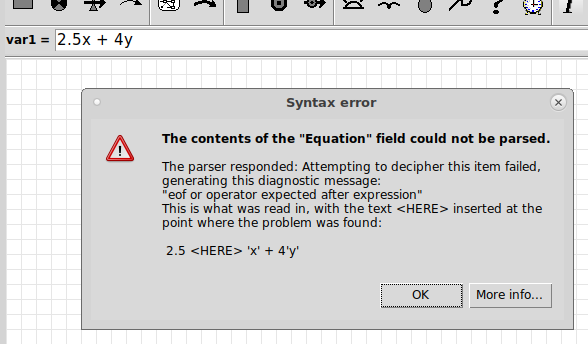# Working with equations : Understanding error messages

## Don't panic!

If you try to set an equation for a component that Simile would not be able to use to generate a value, an error message will appear in a dialogue, something like this:The message is generated by Simile in an attempt to help you find out what is wrong with the equation and how to put it right.

### Syntax errors

There are two basic kinds of error messages. The first is the 'syntax error', an example of which is shown above. This is produced when the equation does not conform to the syntax, or grammar, of the equation language. Often the problem is the result of a typo, but it can also occur when the modeller uses a different idiom for writing an equation than the one Simile understands. For instance, in the above equation, the modeller has written '2.5x' to mean 'multiply x by 2.5', whereas Simile does not recognize this notation -- you need to include a multiplication symbol, as in '2.5*x'.

Simile uses the parser built into its Prolog engine to decode equations, but this takes a slightly different syntax than Simile's equation language, so the text is preprocessed first to make it compatible. In this case, single-quotes have been inserted around the variable names 'x' and 'y'. The parser reports the position at which it found the problem, and the error message shows the preprocessed text with <HERE> inserted at that position. It is usually clear from this what the problem was in the original equation.

Note that only the first syntax error is picked up -- the parser gives up after finding one. So just changing '2.5x' to '2.5*x' in this example will result in another syntax error, because '4y' also needs to be changed to '4*y'.

### Context errors

These are raised if the grammatical structure of the equation makes sense, but there are components that do not correspond to values, operators or functions in Simile, or functions are being used in a type of component in which they make no sense. Each kind of error has its own message, and the explanation should be sufficient to pinpoint the problem.

If you got the error message after clicking 'OK' in the equation dialogue, or hitting Newline or clicking 'tick' in the equation bar, then after you dismiss the message you will be back editing the equation, and you can try to fix the problem. If you get it after leaving the equation bar, you will have to click on the component again and use the pull-down menu to get the equation back into the bar before editing it.

The error message also has a 'More info...' button, although all the info is in fact displayed in the original message. The 'More info...' dialogue does have selectable text that can be copied and pasted for reporting, and a 'Help' button that brings you to this page.